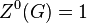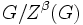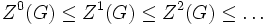Upper central series

(diff) ← Older revision | Latest revision (diff) | Newer revision → (diff)

This article defines a quotient-iterated series with respect to the following subgroup-defining function: center

Definition

The upper central series of a group$G$ is an ascending chain of subgroups indexed by ordinals (including zero), where the$\alpha^{th}$ member is denoted as$Z^{\alpha}(G)$. It is defined as follows:

Case for ordinal$\alpha$ Verbal definition of$\alpha^{th}$ member of upper central series Definition using the$Z^\alpha(G)$ notation$\alpha = 0$ trivial subgroup$Z^0(G) = 1$$\alpha = \beta + 1$ is a successor ordinal. Note that this includes all positive integer values of$\alpha$. its image modulo the previous member is the center of the quotient group by the previous member.$Z^{\alpha}(G)$ is the inverse image of the center of$G/Z^{\beta}(G)$ with respect to the natural projection map$G \to G/Z^{\beta}(G)$. In other words,$\! Z^\alpha(G)/Z^\beta(G) = Z(G/Z^\beta(G))$.$\alpha$ is a limit ordinal. union of all the previous members$Z^\alpha(G) = \bigcup_{\beta < \alpha} Z^\beta(G)$. Equivalently,$Z^\alpha(G) = \langle Z^\beta(G) \rangle_{\beta < \alpha}$

The zeroth member is the trivial group, the first member is the center, and the second member is the second center. In other words:$Z^0(G) \le Z^1(G) \le Z^2(G) \le \dots$$Z^0(G) = 1, \qquad Z^1(G) = Z(G), \qquad Z^2(G)/Z^1(G) = Z(G/Z^1(G)), \qquad Z^3(G)/Z^2(G) = Z(G/Z^2(G)), \dots$$Z^\omega(G) = \bigcup_{n \in \mathbb{N}} Z^n(G)$$\! Z^{\omega + 1}(G)/Z^\omega(G) = Z(G/Z^\omega(G)), Z^{\omega + 2}(G)/Z^{\omega + 1}(G) = Z(G/Z^{\omega + 1}(G)), \dots$

Proof methods

The following articles give a sense of the important methods used to prove facts about the upper central series:

Subgroup properties

Subgroup property Meaning Satisfied? Key facts/examples Restricting to groups with certain properties Relevant proof method
characteristic subgroup invariant under all automorphisms Yes subgroup-defining function value is characteristic -- --
normal subgroup invariant under all inner automorphisms Yes follows from being characteristic and characteristic implies normal -- --
strictly characteristic subgroup invariant under all surjective endomorphisms Yes Combine center is strictly characteristic with strict characteristicity is quotient-transitive -- inductive proof methods for the ascending series corresponding to a subgroup-defining function#Using quotient-transitivity for subgroup properties
quotient-powering-invariant subgroup the subgroup is normal, and if the whole group is powered over a set of primes, so is the quotient. Yes Combine center is quotient-powering-invariant with quotient-powering-invariance is quotient-transitive -- inductive proof methods for the ascending series corresponding to a subgroup-defining function#Using quotient-transitivity for subgroup properties
local powering-invariant subgroup if an element of the subgroup has a unique$n^{th}$ root in the group, that root is in the subgroup. No See second center not is local powering-invariant true for nilpotent groups, see upper central series members are local powering-invariant in nilpotent group. Follows for these from center is local powering-invariant and local powering-invariance is quotient-transitive in nilpotent group inductive proof methods for the ascending series corresponding to a subgroup-defining function#Using quotient-transitivity for subgroup properties
completely divisibility-closed subgroup if the whole group is$p$-divisible for some prime$p$, all$p^{th}$ roots of elements in the subgroup lie in the subgroup. No Follows from center not is divisibility-closed true for nilpotent groups, see upper central series members are completely divisibility-closed in nilpotent group. downward induction on upper central series
fully invariant subgroup invariant under all endomorphisms No Follows from center not is fully invariant No meaningful class of examples. See center not is fully invariant in odd-order class two p-group --

Related subgroup-defining functions

Subgroup-defining function Role in upper central series
center first member, i.e.,$Z^1$
second center second member, i.e.,$Z^2$
hypercenter the final stable member, i.e., the$Z^\alpha(G)$ such that$Z^{\alpha + 1}(G) = Z^\alpha(G)$ ($\alpha$ may be transfinite; however, it must exist).

Related group properties

property meaning in terms of upper central series
Centerless group upper central series stays stuck at trivial subgroup; never gets off the ground
Nilpotent group upper central series reaches whole group in finitely many steps
Hypercentral group transfinite upper central series reaches whole group

Relation with lower central series

For a nilpotent group, the lower central series and upper central series are closely related. They both have the same length, and there is a containment relation between them, which follows from the combination of the facts that upper central series is fastest ascending central series and lower central series is fastest descending central series. However, they need not coincide. Nilpotent groups where they do coincide are termed UL-equivalent groups, and nilpotent not implies UL-equivalent.

Here is a table with some distinctions/contrasts between the two central series:

Nature of fact Fact for lower central series Fact for upper central series
Is the series a strongly central series? Lower central series is strongly central upper central series not is strongly central (i.e., the upper central series need not always be a strongly central series).
What is the nilpotency class of the members of the series? Second half of lower central series of nilpotent group comprises abelian groups, Penultimate term of lower central series is abelian in nilpotent group of class at least three Upper central series may be tight with respect to nilpotency class
Are the members verbal subgroups and/or fully invariant subgroups in the whole group? Lower central series members are verbal (and since verbal implies fully invariant, they are also fully invariant) Upper central series members need not be fully invariant (even for a nilpotent group)